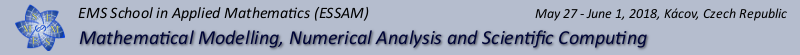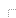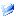HomeESSAMProgramParticipantsTravel InfoVenueOrganizers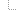Historyphotosflyerprogramscheme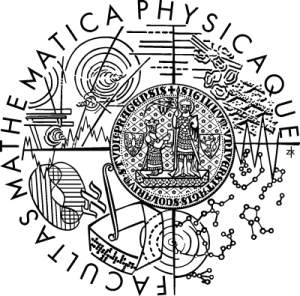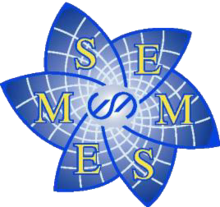Last update:
23.01.2018

# Program

The lectures will be prepared with a broad multidisciplinary audience in mind, and at each school a broad scope, ranging from modeling to scientific computing, will be covered. The four main speakers will deliver a series of three 70-minutes lectures. Ample time within the school is allocated for the promotion of informal scientific discussions among the participants.

## Plenary speakers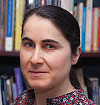Françoise Tisseur The University of Manchester, UK School of Mathematics Alan Turing Building Manchester, M13 9PL
The nonlinear eigenvalue problem

Nonlinear eigenvalue problems (NEPs) arise in many areas of computational science and engineering, including acoustics, control theory, fluid mechanics and structural engineering, and in the past ten years there have been numerous breakthroughs in the development of numerical methods.

In the first lecture, I will present simple but representative examples of NEPs associated with matrix-valued functions that depend nonlinearly on a single parameter. I will discuss some interesting mathematical properties of nonlinear eigenproblems, present their solution structure, including discussions of invariant pairs, Keldysh's theorem, and generalized Rayleigh functionals, as well as some perturbation results.

In the second and third lectures, I will present three classes of numerical methods for NEPs:
1. solvers based on Newton's method, illustrating their convergence properties with examples;
2. solvers using contour integrals, discussing their dependence on parameters;
3. solvers based on the linearization of rational eigenvalue problems. I will discuss practical and efficient construction of rational interpolants via sampling of the matrix-valued function defining the NEP.Nicholas J. Higham The University of Manchester, UK School of Mathematics Alan Turing Building Manchester, M13 9PL
Multiprecision Algorithms

Today's computing environments offer multiple precisions of floating-point arithmetic, ranging from quarter precision (8 bits) and half precision (16 bits) to double precision (64 bits) and even quadruple precision (128 bits, available only in software), as well as arbitrary precision arithmetic (again in software). Exploiting the available precisions is essential in order to reduce the time to solution, minimize energy consumption, and (when necessary) solve ill-conditioned problems accurately.
In this course we will describe the precision landscape, explain how we can exploit different precisions in numerical linear algebra, and discuss how to analyze the accuracy and stability of multiprecision algorithms.

Lecture 1: IEEE standard arithmetic and availability in hardware and software. Motivation for low precision from applications, including machine learning. Applications requiring high precision. Simulating low precision for testing purposes. Software for high precision. Challenges of implementing algorithms in low precision.
Lecture 2: Basics of rounding error analysis. Examples of error analyses of algorithms, focusing on issues relating to low precision.
Lecture 3: Solving linear systems using mixed precision: iterative refinement, hybrid direct-iterative methods. Multiprecision algorithms for matrix functions, focusing on the matrix logarithm.Eduard Feireisl Institute of Mathematics AS CR, Czech Republic Žitná 25 CZ - 115 67 Prague 1
Mathematics of fluids in motion

We discuss solvability of the basic problems of fluid mechanics as the Navier-Stokes and/or the Euler equations. We examine this problem in the context of complete fluid systems. These may be seen as the simplest mathematical model that complies with all basic laws of thermodynamics. We address the question of well and ill posedness with regard to the recent result obtained via a method commonly known as convex integration. We introduce a general class of solutions - the dissipative measure valued solution - that may be seen as the largest class of objects that complies with the principle of weak-strong uniqueness. We discuss several applications of this new concept of solutions, in particular to problems involving singular limits.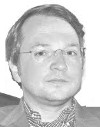Roland Becker Université de Pau et des Pays de l'Adour, France Laboratoire de Mathématiques Appliquées Bâtiment IPRA Avenue de l'Université-- BP 1155 64013 Pau Cedex
Finite element methods for boundary and interface problems

These lectures are concerned with the discretization of boundary value and interface problems by adaptive finite element methods. They are motivated by applications in solid and fluid mechanics, which provide a variety of different boundary conditions, either because of physical modeling or the need to limit the computational domain. In addition, many problems involve different physical materials, which lead to coupling of different partial differential equations by interface problems.
The mathematical theory of finite elements is mostly concerned with approximation properties, stability and error analysis, limiting the considered models to the simplest boundary conditions and generally excluding multi-physical couplings. Therefore, a standard first course on the mathematical theory of finite elements generally focusses on elliptic problems with homogenous Dirichlet data.
In recent time, considerable research has been made to develop numerical methods for multi-physics with an without meshes matching at the interfaces; naturally, non-matching meshes are at least interesting for problems with a priori unknown position of interfaces or dynamically moving interfaces.
These notes focus on finite element methods based on weak treatment of interface couplings. This can in principle be achieved by means of Lagrange multipliers. At least from a computational point of view, methods avoiding multipliers are preferable, since they avoid discretization of the multiplier spaces and do not require additional work for iterative solution of the resulting systems.
An important additional property of weak coupling is the possibility of weighting contributions from different partial differential operators involved in the system. Thereby it is possible to establish a discrete weak formulation which makes sense for the limit of a singularly perturbed problem, such as the convectiondiffusion problem with vanishing viscosity parameter.
For more details we refer to abstract.